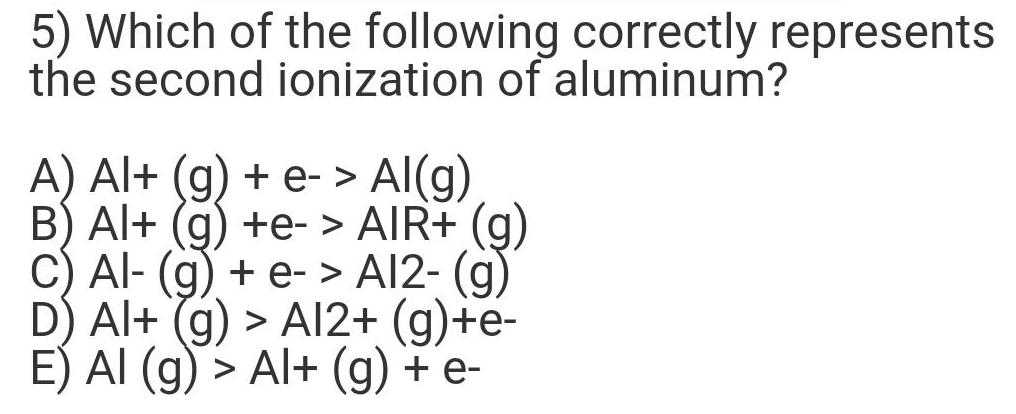Question:

# Which of the following correctly represents the second

Last updated: 7/31/2022Which of the following correctly represents the second ionization of aluminum? A) Al+ (g) + e-> Al(g) B) Al+ (g) +e-> AIR+ (g) C) Al- (g) + e- > A12- (g) D) Al+ (g) > A12+ (g)+e- E) AI (g) > Al+ (g) + e-